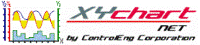XY Chart NET 3 Control Reference
AbsMaxYValue Property

Use this property to return the absolute maximum y-value of a specific y-axis.

Syntax
 [Visual Basic] ReadOnly Property AbsMaxYValue(YAxisNumber As Integer) As Double [C#] double XYChartNETCtl.get_AbsMaxYValue(int YAxisNumber) [C++] property double AbsMaxYValue[int]

Parameters
YAxisNumber
The y-axis index to obtain the absolute maximum value of.  Valid values are 0..(NumYScales - 1).
Remarks

Absolute maximum is the highest value that is displayed on the graph for the specified y-axis. The value returned is of type Double.This property is read-only and should only be called after a Refresh call has been made.
Example
```' XYChartNETCtl1 is the name of the XY Chart NET control instance placed on the form.

Dim idx As Integer
Dim tmpMin As Double
Dim tmpMax As Double

For idx = 0 To XYChartNETCtl1.NumYScales - 1
tmpMin = XYChartNETCtl1.AbsMinYValue(idx)  'Get the absolute min value for the specified y-axis
tmpMax = XYChartNETCtl1.AbsMaxYValue(idx)  'Get the absolute max value for the specified y-axis
Next idx
```
```// XYChartNETCtl1 is the name of the XY Chart NET control instance placed on the form.

double tmpMin;
double tmpMax;

tmpMin = XYChartNETCtl1.get_AbsMinYValue(1);  //Get the absolute min value for y-axis 1
tmpMax = XYChartNETCtl1.get_AbsMaxYValue(1);  //Get the absolute max value for y-axis 1
```
```// XYChartNETCtl1 is the name of the XY Chart NET control instance placed on the form.

double tmpMin;
double tmpMax;

tmpMin = XYChartNETCtl1->AbsMinYValue;  //Get the absolute min value for y-axis 0
tmpMax = XYChartNETCtl1->AbsMaxYValue;  //Get the absolute max value for y-axis 0
```